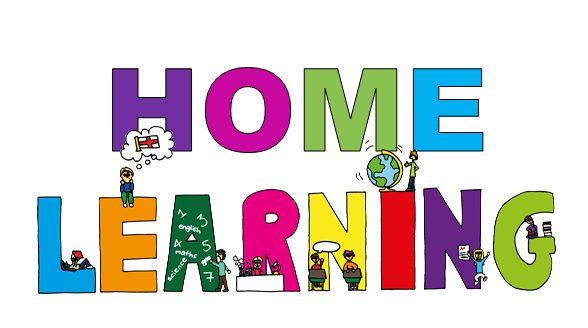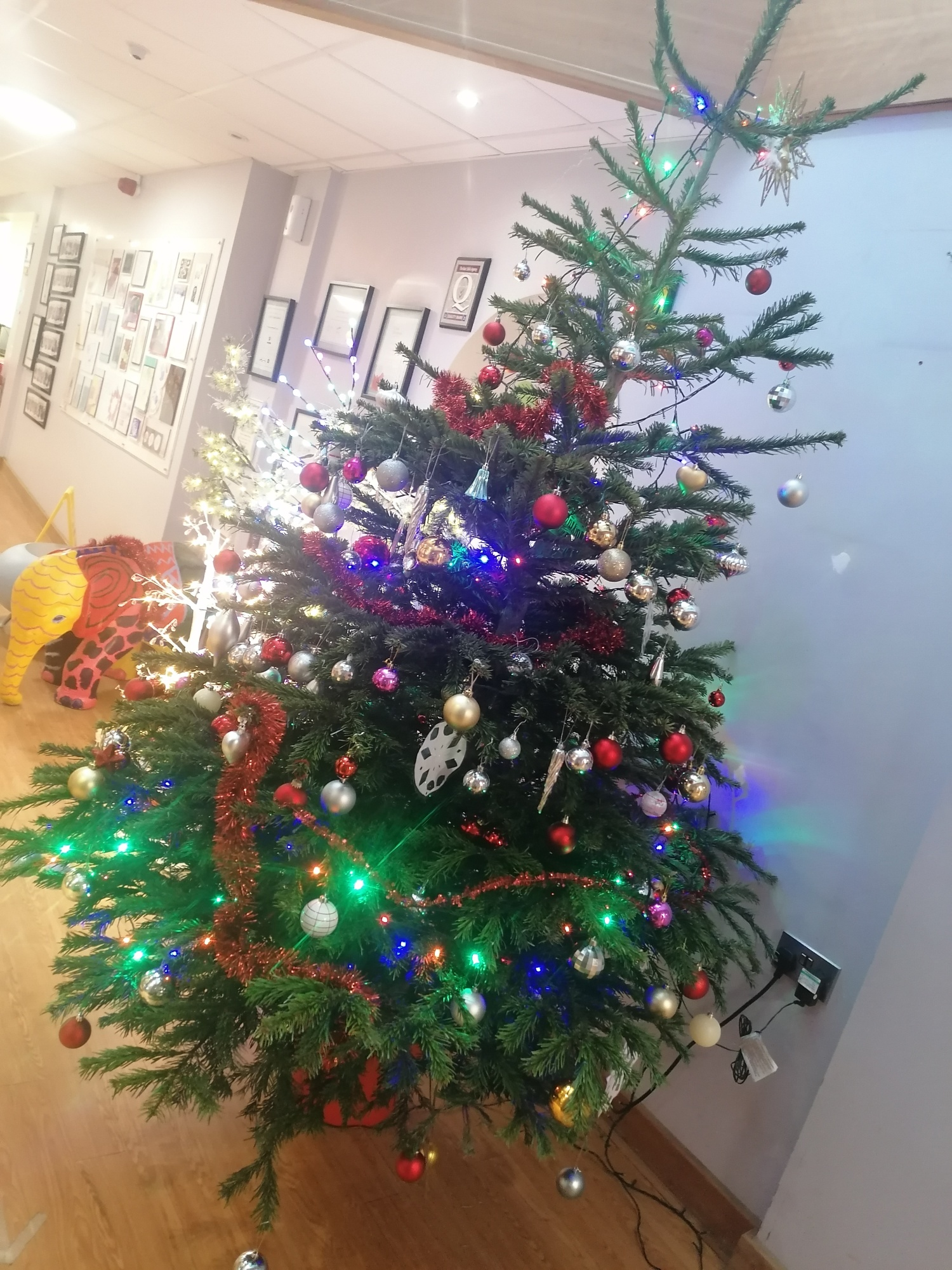Christmas Home LearningEnglish

Lesson 1

To listen to and join in with an explanation

In this lesson, we will meet Dr Explanation, who will explain why the king was amazed in the Talking Sweet Potato story. We will start to use the special word- because.

Lesson 2

To map and speak an explanation

In this lesson, we will create a text map for the explanation and then talk the map using the word because.

Lesson 3

To step and speak an explanation

In this lesson, we will step out the explanation to help us learn it.

Lesson 4

To sing an explanation

In this lesson, we will explore the explanation through song.

Maths

Lesson 1

Recognising the value of one penny and the values of other coins

In this lesson, we will learn the value of one penny and the other coins.

Lesson 2

Exploring different combinations of coins for a given total of up to 10p

In this lesson, we will be exploring different combinations of coins to give a total amount up to 10p using a ten and five frame.

Lesson 3

Exploring different combinations of coins with a total of up to 20p

In this lesson, we will be exploring different combinations of coins to give a total amount up to 20p using a number track.

Lesson 4

Exploring giving change from ten pence

In this lesson, we will be exploring giving change from ten pence using a ten frame.

English

Lesson 1

To listen and respond to a set of instructions

In this lesson, we will be listening to a set of instructions in role about how to make a magic hat and answering questions to check our understanding.

Lesson 2

To tell a set of instructions from memory

In this lesson, we will be learning to tell a set of instructions from memory using a four step process - Hear, Map, Step, Speak.

Lesson 3

To role play a set of instructions

In this lesson, we will be using role play to give instructions clearly and precisely.

Lesson 4

To use imperative verbs

In this lesson, we will be using imperative verbs to give clear and precise instructions.

Maths

Lesson 1

Revisiting the value of coins: Comparing value

Today, we are going to recognise and compare the value of coins

Lesson 2

Coins and notes

Today we will learn to recognize the value of coins and notes and learn how to use the symbol for pound accurately

Lesson 3

Counting money in a set of coins

Today, we will learn how to find the total value of money in a set of coins

Lesson 4

Revisiting the value of coins

Today we will learn how to use different coins to make the same amount.

English

Lesson 1

To explore simple and compound sentences

In this lesson, we will revise our understanding of simple and compound sentences.

Lesson 2

To revise our understanding of simple and compound sentences

In this lesson we will revise our understanding of simple and compound sentences.

Lesson 3

To explore complex sentences

In this lesson, we will explore 'As' complex sentences and have a go at writing our own, based on the clip we watched in the first lesson.

Lesson 4

To develop understanding of complex sentences

In this lesson, we will explore using 'As' adverbial complex sentences and complex sentences that have relative clauses in them. We will practise writing sentences throughout the lesson.

Maths

Lesson 1

To identify and recognise angles

In this lesson, we will start to understand what the word ‘angle’ means. We will begin to identify angles within objects and shapes before we start to compare the size of angles.

Lesson 2

To identify angles inside 2D shapes

In this lesson, we will review what an angle is. We will begin to explore various angles that are found inside 2-D shapes before noticing patterns between the number of sides and the number of angles.

Lesson 3

To recognise right angles

In this lesson, we will be building on your angles learning and focusing specifically on right angles. We will explore these on their own, in shapes and in everyday objects.

Lesson 4

To recognise obtuse and acute angles

In this lesson, we will be building on our angle knowledge by introducing the terms acute and obtuse for those angles which are smaller (acute) and greater (obtuse) than right-angles. We will have time to look at these angles separately and within different shapes.

English

Lesson 1

To explore the function of apostrophes

In this lesson, children will review the function of apostrophes before identifying how apostrophes have been used in different examples.

Lesson 2

To explore subject, verb, object

In this lesson, children review word class in a sentence before reviewing knowledge of subject, verb and object. Following this, children practise identifying the subject, verb and object in a sentence, before writing their own sentence and identifying the subject, verb and object.

Lesson 3

To develop a rich understanding of words associated with working hard

In this lesson, we will introduce new vocabulary, identify word pairs and synonyms and apply the vocabulary in sentences.

Lesson 4

To explore the functions of a colon

In this lesson, we will practise using parentheses in the three forms (brackets, dashes and commas). Then we will explore why we use colons in writing and its uses. Each use will be looked at in more detail and then children will get to put their knowledge of the different uses into practice.

Maths

Lesson 1

Solve problems involving the calculation of percentages of amounts

In today’s lesson, we will be learning to find a percentage of an amount, including using efficient strategies and looking at the link between percentages and fractions.

Lesson 2

Solve problems involving the use of percentages for comparison

In this lesson, we will be solving problems involving the use of percentages for comparison.

Lesson 3

Interpret Mean as an Average

In today’s lesson, we will explore averages before creating a formula for calculating the mean. We will then move on to find missing values using the mean.

Lesson 4

Interpret Line Graphs

In today’s lesson, we will explore discrete and cumulative data and interpret different types of line graphs.

KS1

Identifying tempo: Changes in tempo

In this lesson, we will be learning about tempo (speed). We will learn the different Italian terms for fast and slow and identify changes in tempo.

KS2

Understanding pulse and rhythm

In this lesson, we are going to find the pulse and explore rhythm.